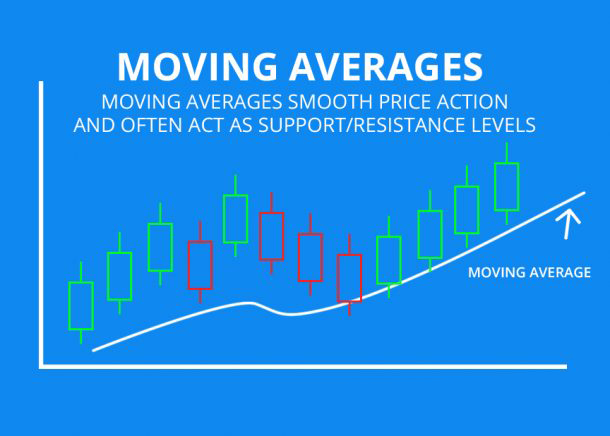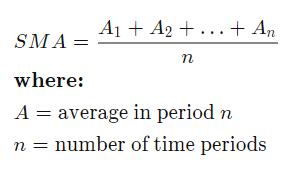# What are Moving Averages?

0
271Moving Averages is the graph of the average closing price for a number of days in the past. It could be 5 days, 30 days, 60 days, or even more. Almost all charting applications will have the option to draw these automatically.

The two basic and commonly used moving averages are the simple moving average (SMA), the exponential moving average (EMA)
Simple moving average is the simple average of a security over a defined number of time periods while
Exponential moving average gives greater weight to more recent prices.The simple moving average calculates the arithmetic mean of a security over a number (n) of time periods, A.

## Significance of Moving Averages

Moving averages lag behind current price action because they are based on past prices; the longer the time period for the moving average, the greater the lag. Thus, a 200-day Moving Average will have a much greater degree of lag than a 20-day moving average because it contains prices for the past 200 days. The 50-day and 200-day MAs are widely followed by traders, with breaks above and below this moving average considered to be important trading signals.